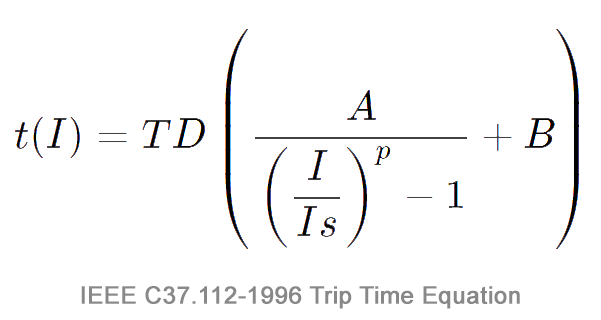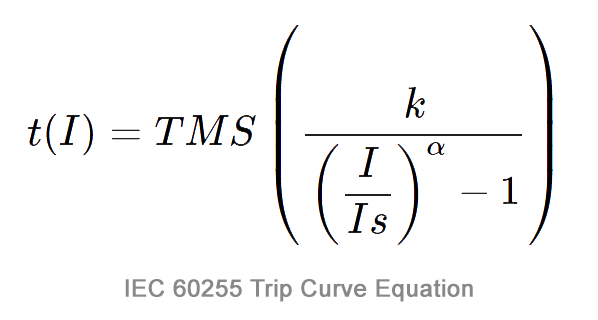# Inverse Time Overcurrent Relays and Curves Explained

Overcurrent relaying is one of the most simple and economical types of protection used for power system feeders, transformers, generators and motors. Since the advent of simple electromechanical elements, to advanced microprocessor applications used in modern relays, overcurrent protection has been at the heart of the power system for centuries.

The characteristics of overcurrent relays are based on operating times typically governed by a time vs. current curve. There are three main types of overcurrent relay: (1) Instantaneous, (2) Time-Dependent (Definite time or inverse), and (3) Mixed (Definite time and Inverse).

1. Instantaneous relays operating time are usually less than 3 cycles. These relays operate without an intentional time delay, so they are referred to as instantaneous units. The pickup current is adjustable and the application engineer can choose various settings from a wide range.

2. Time-dependent relays, as the name implies, operate with an intentional time delay. The minimum current at which the relay operates (pick up current) and time before trip are both adjustable.

There are a total of five types of time dependent relay, broken into two categories: Definite Time and Inverse Time.

A. Definite-time relays operate with some intentional time delay and is adjustable along with the current pickup level. Although these relays are adjustable, their time delays are not necessarily dependent on the current value.

B. Inverse-time relays have an operating time depending on the magnitude of the current, generally with an inverse characteristic (the operation time of the relay is smaller as the current gets larger). These relays also have two settings: the pick-up current and the curve type.

In electromechanical relays the curve is set by means of a dial which is why the setting is referred to as the “time dial setting” or TDS. In some relays, a Time Dial Multiplier is used instead of Time Dial setting but their functions are similar.

## TOC & IDMT

Inverse Time Over Current is also referred to as Time Over Current (TOC), or Inverse Definite Minimum Time (IDMT) which indicates that the trip time of the relay is inversely proportional to the applied fault current.

Overcurrent Relay Time Delay Curve Types

There are a total of five types of time dependent relay, broken into two categories: Definite Time and Inverse Time.

The trip time of an inverse curve is calculated from the following parameters:

• Trip curve. Selected from the standard set of IEC and IEEE curves.
• Relay pickup current (A). The electrical current pickup set point Is in the relay.
• Fault current (A). The expected short circuit fault current I.
• TMS or TD setting. IEC time multiplier setting (TMS). IEEE time dial (TD).

## Degrees of Overcurrent Inverse Curves

How long it takes for the relay to trip will vary depending on the curve slope, these curves can be used by engineers to coordinate with other protective devices upstream for selectivity and backup. There are five different types of time overcurrent relay according to American standards.

Their time-current characteristic curves are:

• Definite minimum (CO-6)
• Moderately inverse (CO-7)
• Inverse (CO-8)
• Very inverse (CO-9)
• Extremely inverse (CO-11)

Degrees of Overcurrent Inverse Curves

When plotted on a chart, the different characteristics between each curve become apparent. The more inverse the curve shape, the greater difference in trip times.

Electromechanical overcurrent relays are often constructed to a specific curve shape, such as the ABB CO-6, CO-7, CO-8, ect. To change the EM curve shape means to replace the whole unit, which can be very expensive and results in surplus equipment.

Modern digital relays are programmable and thus curve shapes can easily be changed without the need for replacement.

## How Inverse Time Curves are Calculated

Each standardized relay protection curve will have its trip time calculated from either IEEE C37.112 or IEC 60255 equations.

### IEEE C37.112-1996 Equation for Trip TimeIEEE C37.112-1996 Equation for Trip Time

• A = Time factor for over-current trip
• I = Actual Current
• Is = Relay Pickup Setting
• p = Exponent for inverse-time
• B = Timer coefficient for over-current trip
Note: IEEE C37.112-1996 does not specify coefficients in their standard curve equation and therefore each manufacturer’s curve is similar. A TDM (Time Dial Multiplier) is sometimes used instead of TD (Time Dial). The relationship is: TDM = TD / 7
Curve type A B p
Moderately inverse 0.0515 0.114 0.02
Very inverse 19.61 0.491 2.0
Extremely inverse 28.2 0.1217 2.0

### IEC 60255 Equation for Trip TimeIEC 60255 Equation for Trip Time

• Is is the current setting.
• I is the actual current.
• k and α are the curve type constants.
Curve type k α
Standard inverse 0.140 0.020
Very inverse 13.5 1
Extremely inverse 80 2
Long time standard inverse 120 1

### Examples

A. Calculate the tripping time for a relay set at 1000A pickup current and TMS setting of 1 (IEEE Very Inverse) with 10kA of fault current.

• 10000 / 1000 = 10
• 10^2 = 100
• 100-1 = 99
• 19.61 / 99 = 0.198
• 0.491 + 0.198 = 0.689

0.689 Seconds

B. Calculate the tripping time for a relay set at 1000A pickup current and TMS setting of 1 (IEC Very Inverse) with 10kA of fault current.

• 10000 / 1000 = 10
• 10^1 = 10
• 100-1 = 9
• 13.5 / 9 = 1.5

1.5 Seconds

## Mixed Curves (Definite time and Inverse) Overcurrent Relays

Thanks to the advent of the digital relay, its possible to have all of the advantages that come with the different types of relay elements packed into a single programmable unit.

Nearly any combination of instantaneous, definite-time, and inverse-time elements may be used. The most common type of mixed relay is the inverse definite with minimum time lag relay (IDMT) which combines inverse characteristic plus definite time characteristics.

Microprocessor-Based (Digital) Relays. Photo: TestGuy.